## Sunday, 1 April 2012

### Class 10 - Science - Ch12 - Electricity - Q & A

Electricity

Q & A

Q1: What type of charge does an ebonite rod acquire when it is rubbed with wool? What is
the nature of the charge acquired by wool?

Answer: Ebonite rod has a negative charge and woollen cap has an equal amount of positive charge.

Note: Charging by friction always produces equal and opposite charges on the twobodies which are rubbed in intimate contact.

Q2: What is the charge on an electron (e)?

Answer: -1.6 x 10 -19 Coulombs (C)

Q3: Define Coulomb's law.

Answer: The magnitude of the force of attraction (or repulsion) between two point charges is
directly proportional to the quantity of charge present on each of them and inversely
proportional to the square of the distance separating them.

Let q1 and q2 be two like charges separated by a distance d, then the two charges will continue to repel each other with a force (F). Mathematically it is represented as:

Q4: What is the SI unit of charge? Is it a scalar or vector quantity?
Answer: SI unit of charge is Coulomb (C). It is a scalar quantity.

Q5: Define 1C or one coulomb.

Answer: 1C charge is the charge which when placed at a distance of 1 m from an equal like charge in a vacuum, experiences a repulsive force on 1N.

Q6: How many electrons make one coulomb?

Let us assume, n number of electrons constitute 1C.

Charge on 1 electron = -1.6 x 10 -19 C
∴ total charge Q = 1C = n x |e|
i.e. n = Q/|e| = 1/ 1.6 x 10 -19= 6.25 x 10 18 electrons        ...(answer)

Q7: What are the fundamental laws of Electrostatics?

• There are two types of charges namely positive and negative.
• Like charges repel each other and unlike charges attract each other.

Q8: Define current

Answer: The rate at which charges move in a conductor is called electric current i.e. quantity of charges crossing a point in unit time.
I = q/t
where I is current in amperes (A)
q is total charge in Coulombs (C)
t - denotes time in seconds (s).

∴  1 A = 1 C/s

Q9: Is Ampere scalar or vector?

Q10: Two objects, with charges are 1.0 and 1.0 C, are separated by 1.0 km. Find the magnitude of the attractive force that either charge exerts on the other.

Answer: Given q1 = 1.0 C, q2 = 1.0 C, distance d = 1.0 km = 1.0 x 103 m
Applying coulomb's law,

F = (9 x 109 x 1.0 x 1.0) / (1.0 x 103 ) = 9 x 103 N

Q11: Define Electric Potential.

Answer: It is  the work done in carrying a unit positive charge from infinity to that point against any electric field. it is denoted by symbol V. Its SI unit is volt.
i.e. V = W/ q

Q12: Define electric potential difference.

Answer: When a unit charge ( = 1 C) is carried from one point to another point, the work done is called potential difference.
E.g. potential difference between A and B is:

VAB = W  (Work done to carry charge q from A to B)  ÷ q (Charge in Coulombs)

The SI unit of potential difference is Volt (V).
1 Volt = 1 Joule / 1C

Q13: How many electrons per second pass though a given point in a wire carrying 2.0 Amps?

Given Current (I) = 2.0 A
Time t = 1.0 s
I = q/t  ∴ q = I x t = (2.0)(1.0) = 2.0 C
Since Q = n x e (where n is number of electrons and e = 1.6 x 10 -19 C)
∴  n = 2.0 ÷  1.6 x 10 -19= 1.25 x 10 19            ...(Answer)

Q14: A charge of 2C moves between two plates, maintained at a potential difference of 1V. What is the energy acquired by the charge?

Given Potential Difference (V) = 1V
Charge (q) = 2 C

Q15(NCERT): Name a device that helps to maintain a potential difference across a conductor.

Answer:  Cell, battery, power supply, etc.

Q16: Define electric circuit. Will the electric current flow in
(a) an open circuit
(b) a closed circuit

Answer: A continuous and closed path of an electric current is called an electric circuit.

(a) Open Electric Circuit:  In an open electric circuit, the electric current does not flow as there is
some break in the path, i.e., either the key is open or some other end is not connected.

(b) Closed electric circuit. In this an electric current flows as the path is closed.

Q17: A current of 0.75 A is drawn by a filament of an electric bulb for 5 minutes. Find the amount of electric charge that flows through the circuit.

Answer: Given, Current (I) = 0.75 A
duration (t) = 5 min = 5 x 60s = 300 secs

Since, Q = I x t = 0.75 x 300 = 225 C ... (answer)

Q18: How much work is done in moving a charge of 5 C across two points having a potential difference 20 V?

Given here,
Charge (q) = 5 C
Potential Difference (V) = 20 V
Work done in moving a charge W = qV = 5 x 20 = 100 J  ... (answer)

Q19: Define resistance. What is the SI unit of resistance?

Answer: Resistance is a property that resists the flow of electrons in a conductor. It controls the magnitude of the current. The SI unit of resistance is ohm (Ω).

Q20: To make an uncharged object have a negative charge we must:
b. remove some atoms
d. remove some electrons

Choose the appropriate option.

Q21: State Ohm's law.

Answer: Under similar physical conditions and at constant temperature, the current flowing through a wire is directly proportional to the potential difference applied across its ends.

⇒ I ∝ V               (where I is current and V is potential difference)
⇒  V/I = constant = R (where R is the resistance offered.)
⇒ V = IR
⇒ R = 1Ω = 1V/1A

Graphically it can be shown as:
Ohm's law is applicable to metallic conductors only.

Q22: What factors affect the resistance?

Answer: Resistance of the conducting wire depends on:
• Nature of material (called resistivity ρ)
• directly proportional to the length of the wire (l) i.e. R ∝ l
• inversely  proportional to the cross sectional area of the wire (A)  i.e. R ∝l/A
• varies with temperature.
Mathematically,  for metal conductors at fixed temp.
R =  ρl/A

Q23: What is dangerous "High Voltage" or "High Current"?

Answer: It is high level of current is dangerous. A current of 1mA (milli Ampere) gives you a tingling effect. Current above 10mA is painful. Current of value 12-20mA can paralyze muscles and current above 100mA can collapse the heart.
You might have seen 'Van de Graaf generator' at Science Museum (see figure below: source: http://www.mtu.edu/news/images/2010/image29764-horiz.jpg)Van de Graaf Generator (source: Michigan Tech University)The Van de Graaf generator creates thousands of volts but current is less than 1mA (enough to tingle and raise you hair).
You may have seen 'High Voltage' sign boards near transformers, Metro Stations.
Generally the wires carrying high voltage at these places do carry high current (greater than 1 Amperes) which can produce lethal effects on your body.

Q24: If R1 is 20 Ω, and R2 is 40 Ω, what is the current flowing in the circuit if the two resistors are connected in series. The battery connect is 6.0 V?

Answer: When resistors are connected in series, effective resistance Req = R1 + R2
i.e. Req = 20 + 40 = 60 Ω
Applying Ohm's law,
I = V/Req = 6 / 60 = 0.1 A             ...(answer)

Q25: What is current resistance in each resistor  for a parallel combination of 3.0 Ω, 3.5 Ω and 4.0 Ω resistors. The battery connected is of 8V. Also find the effective resistance and effective current.

Q26: Why an ammeter will burn out if connected in parallel in an electric circuit?

Answer: Ammeter has very low resistance. If connected in parallel, a large current will draw, may be enough to burn it up.

Q27: Name the instrument used to change the resistance of the circuit.

Q28: A cylindrical wire has a radius R and length L. If both R and L are doubled, the resistance of the wire
(a) increases
(b) decreases
(c) remains the same.

(Hint: Use equation R =  ρl/A, A will be come 4 times).

Q29: Find the equivalent resistance for the following circuit when

(a) Switch is closed.
(b) Switch is open.

Answer: Given, R1 = 10Ω, R2 = 5Ω,  R3= 5Ω and R4 = 10Ω
Case a: When switch is closed. R1 and R3 will be in parallel. Similarly R2 and R4 are in parallel (as the following figure shows).
These two parallel resistors are in series. i.e. (R1 || R3) + (R2 || R4).
i.e. 1/R13 = 1/R1 + 1/R3 = 1/10 + 1/5 = 3/10 ⇒ R13 = 10/3 Ω
Similarly R24 = 10/3 Ω

R(eqv.) = R13 + R24 = 10/3 + 10/3 = 20/3 Ω  ...(answer)

Case b: When switch is open, as shown in below figure, R1 and R2 will be series. Similarly, R3 and R4 will be series. And these two series circuits are connected in parallel to each other.
∴ R12 = R1 + R2 = 10 + 5 = 15Ω
Also R34 = R3 + R4 = 5 + 10 = 15Ω

1/R(eqv.)  = 1/R12 + 1/R34 = 1/15 + 1/15 = 2/15 Ω
R(eqv) = 15/2 Ω = 7.5 Ω ...(answer)

Q30(): Consider the following circuit consisting of infinite resistors of value R. Compute the equivalent resistance RAB between A and B.
Answer: No doubt the problem is beyond CBSE syllabus :)
However, If the same problem is represented in equation form (Class 10 Maths - Quadratic Equations, recurring rational numbers), you can easily solve this.

Let RAB be the equivalent resistance. If we chop the first two resistors R, still the remaining circuit represents  RAB
The circuit can be represented as:
Let us assume R = x.

Q 31: State True or False
(a) Current gets divided in series combination.
(b) 1 Watt = 1 Volt / 1 Ampere
(c) Tungsten is used for making bulb elements.
(d) The graph between V and I for metals is a curve.
(e) Insulators have high resistivity and negligible conductivities.
(f) Metals and alloys have low resistivity in the range of 10-8 Ωm to 10-16Ωm.

(a) False
(b) False
(c) True
(d) False
(e) True
(f) True

Q32: Why is the series arrangement not used for domestic circuits?

Answer: Series arrangement is not used for domestic circuits because of the following reasons:
• If one appliance gets fused, it will break the circuit and all other appliances will stop working.
• In series, the voltage drops at each appliance. No appliance would be working at required voltage.

Q33: Why are alloys commonly used in electrical heating devices?

• Alloys have high resistivity.
• They do not oxidize easily.

Q34: Why are bulbs filled with chemically inactive Nitrogen or Argon gas.

Answer: It is done in order to prevent oxidation of Tungsten filament.

Q35: State thermal effect of electricity.

Answer: When current is passed through a conductor it gets heated up. It is called thermal effect.
If heat produced is H joules, then
H α potential difference (V),
H α current flowing
H α duration (time) the current flows.

H α VIt or H = kVIt

Taking k = 1, V = 1 volt, I = 1A and t = 1 second, H = 1J.

H = VIt
Applying Ohm's law (V = IR),

H = VIt = I2Rt = V2t/R

H =  I2Rt is also known as Joule's law of heating.

Q36: A potential difference of 250V is applied across a resistance of 1000 ohm. Calculate the heat energy produced in the resistance in 10 s.

Answer: Given V = 250 volts, R = 1000 Ω t = 10s
H = V2t/R = 250 x 250 x 10/1000 = 625 J ... (answer)

Q37: Judge the equivalent resistance when the following are connected in parallel
(a) 1 Ω and 106 Ω,
(b) 1 Ω and 103 Ω, and 106 Ω.

(a) 1 Ω and 106 Ω connected in parallel
1/R = 1/1 + 1/106
⇒ R = 106/(106+ 1)  ≈ 106/106 = 1 Ω.         ...(answer)

(b) 1 Ω and 103 Ω, and 106 Ω. connected in parallel.
1/R = 1/1 + 1/103 + 1/106
⇒ R = 106/(106+ 103 +1)  = 106/1001001 = 0.99900 Ω  ... (answer)

Q38: Will current flow more easily through a thick wire or a thin wire of the same material, when connected to the same source? Why?

Since Resistance(R) is inversely  proportional to the cross sectional area of the wire (A)  i.e. R ∝l/A. Thicker the wire, lesser will be the resistance. Therefore, current will flow more easily in a thick wire as compared to a thin wire of same material and length.

Q39: An electric iron has a rating of 750W, 220V. Calculate
(i) current passing through it, and
(ii) its resistance, when in use

Power(P) = 750W
V = 220 volts
P = VI  ⇒ I = P / V = 750 / 220 = 3.4 A   ...(answer)
R = V/I = 220/3.4 = 64.7Ω  ...(answer)

Since the resistance of the conductor is inversely proportional to its area of cross-section. Therefore thicker wire will draw more current and resistance will be less.

Q40: What is the commercial unit for electrical energy?

Answer: Killowatt hour (KWh) is the commercial unit for electrical energy.
1 KWh = 1000 W hour -= 1000 Joules/second x 3600 second
i.e.      = 3.6 x 106 Joule.

Q41 (CBSE): In a household, an electric bulb of 100W is used for 10 hours and an electric heater of 1000W is used for 2 hours. Calculate the cost of using bulb and the heater for 30 days. Take the cost of one unit of electrical energy as 2 rupees.

Answer:  Energy Consumed = Power x time
∴ Energy consumed in 30 days will be
= energy consumed by bulb (E1) + energy consumed by heater (E2)
E1 = 100 x 10 x 30 = 30000 Wh = 30KWh
E2 = 1000 x 2 x 30 = 60000 Wh = 60KWh

Total energy consumed = 30 + 60 = 90KWh
Cost of electrical energy = 2 x 90 = INR 180           ...(answer)

Q42: An electric fuse used in households, works  on which principle?

Answer:  Electric fuse works on the principle of Joule’s heating.

Q43: How does electric fuse works?

Answer: Electric fuse works on the principle of Joule’s heating. It protects circuits and appliances by stopping the flow of any unduly high electric current. The fuse is placed in series with the device. It consists of a piece of wire made of a metal or an alloy of appropriate melting point, for example aluminium, copper, iron, lead etc. If a current larger than the specified value flows through the circuit,
the temperature of the fuse wire increases. This melts the fuse wire and breaks the circuit.

Q44: How electric fuse is connected with electrical devices i.e. in parallel or in series?

Q45: Which type of material is commonly used for fuse wire?

Answer: It consists of a piece of wire made of a metal or an alloy of appropriate melting point, for example aluminium, copper, iron, lead etc.

Q46: Why is electric power transmitted over long distances at high voltage?

Answer:  It is done to minimize power losses due to Joule's heating. We know that Power = V ✕ I and Heat generated  = I2Rt. Thus by keeping low current, heating effect is reduced and high voltage retains the total power.

Q47(CBSE):  A piece of wire having resistance R is cut into four equal parts.

a. How does the resistance of each part compare with the original resistance?

b. If the four parts are placed in parallel, how will the resistance of combination compare
with the resistance of original wire?

Answer: (a) Since R ∝ length of wire. Decrease in length will reduce the resistance. Each piece will have resistance = R/4Ω = 0.25RΩ

(b) In parallel, the effective resistance will be R/16Ω

Q48(CBSE 2011): Why do the wires connecting an electric heater to the mains not glow while its heating element does?

Answer: The heating element of an electric heater is a high value resistor. The amount of heat produced by it is proportional to its resistance. The resistance of the element of an electric heater is very high. As current flows through the heating element, it becomes too hot and glows red. On the other hand, the resistance of the cord (wire connecting heater to mains) is low. It does not oppose the flow of current and hence it does not become red when current flows through it.

Q49: Electrical resistivity of some substances at 20°C are given below:
Silver = 1.60 × 10-8 Ω m
Mercury = 94.0 × 10-8 Ω m
Ebonite = 1015 - 1017 Ω m
(i)  Which among the above three is a better conductor?
(ii) Which among the above three can be used for making electrical plugs?

Answer: (i) Silver has the lowest resistivity, it is a better conductor.
(ii) Ebonite has very high resistivity. It can be used to make electric plugs.

Q50: What will be the length of a Nichrome wire of resistance 5.0 Ω, if the length of similar wire of 120 cm has a resistance of 2.5 Ω?

Answer: Since Resistance is directly proportional to the length of the wire i.e. R ∝l
⇒ R1/l1 = R2/l2
Given  R1= 5 Ω,    l1 = ?
R2= 2.5 Ω,    l2 = 120 cm
⇒ l1 = R1× l2/R2 = 5 × 120/2.5 = 240 cm

Q51(HOTS): Is Ohm's law a fundamental law? State the reasons.

Answer: Ohm's law is not a fundamental law since it is valid for metallic conductors which show a linear relationship i.e. I α V or R is constant. Ohm's law does not hold good for semiconductor devices since the V-I relationship is non-linear. When current flow in semiconductor devices, due to motion of electrons and collisions, resistance of these devices decreases, thus exhibiting a non-linear behaviour.1.2.amazing these helped me a lot thank you very very much
but some definitions are out of course

3.Good collection of questions

4.5.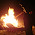nice and amazing questions at some places the units are wrong but no worries keep u the gud work

6.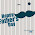very nice set of questions

7.8.I want to take printout of these questions, is it possible? if yes then plz tell how to do it?

1.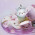yes press ctrl and p at same time

9.SOME OUESTIONS ARE NOT THERE

10.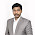11.IB Higher Level Physics | IB Standard Level Physics

12.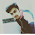13.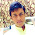Can I get a detailed solution of question 30

14.This comment has been removed by the author.

15.This comment has been removed by the author.

1.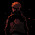This comment has been removed by the author.

16.given questions are good for exams to practice
thanks

17.This comment has been removed by the author.

18.19.good it helped me for my exams

20.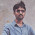Still Problems must be included as they are v.imp..

21.Still Problems must be included as they are v.imp..

22.Good.
How can i be the member of this ?

23.nice questions and very much helpful ,
thanks but you should students to get it copy

24.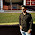very good educational site

25.FABULOUS questions and answers !I LIKE IT:)

26.very good collection of questions and good explanation of answers...